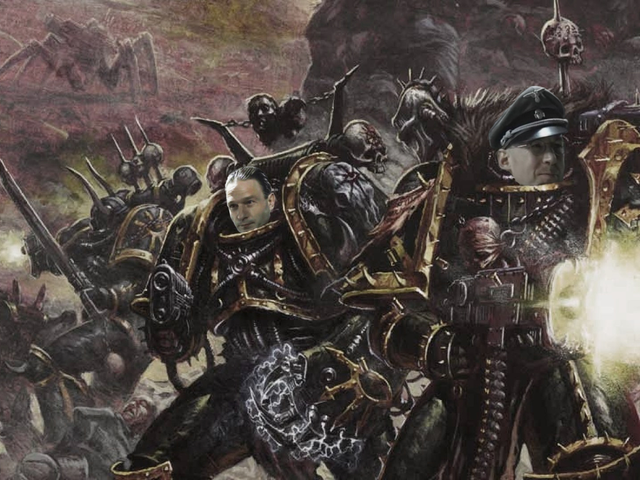# How is discrete mathematics used in games?

## Understanding Discrete Mathematics

In this section, we will delve into the basics of discrete mathematics. Discrete mathematics is a branch of mathematics that deals with objects that can be counted, like whole numbers. Unlike calculus, which deals with continuous numbers, discrete mathematics focuses on distinct, separated values. This field of study has a wide range of applications, especially in computer science, where the data is often in the form of discrete values.

## The Link Between Discrete Mathematics and Gaming

Discrete mathematics and gaming are more interconnected than you might think. At the heart of many video games are algorithms and data structures, both of which are studied extensively in discrete mathematics. This makes discrete mathematics an essential tool for game developers, who use it to solve complex problems, optimize solutions, and create engaging gaming experiences.

## Game Design and Discrete Mathematics

Game design is one area where discrete mathematics is heavily used. Game designers use concepts from discrete mathematics to create game rules and mechanics, create balanced scoring systems, and even design levels. For instance, graph theory, a subfield of discrete mathematics, can be used to model and analyze game maps and levels.

## Algorithms in Game Development

Algorithms, a core concept in discrete mathematics, are vital in game development. They are used to determine how non-player characters (NPCs) make decisions, how game physics work, and how graphics are rendered. Understanding and applying algorithms can help game developers create games that are more engaging, realistic, and fun to play.

## Discrete Mathematics in Game Physics

Discrete mathematics also plays a crucial role in game physics. For instance, discrete mathematics can be used to model and simulate physical phenomena like collisions, gravity, and lighting. This helps game developers create games that look and feel realistic, enhancing the overall gaming experience.

## Graph Theory in Pathfinding

Graph theory, a branch of discrete mathematics, is widely used in pathfinding algorithms in video games. These algorithms help non-player characters (NPCs) find the shortest or most efficient path from one point to another. This is crucial in games where NPCs need to navigate around obstacles and terrain.

## Discrete Mathematics in Game Logic

Game logic is another area where discrete mathematics is extensively used. Game logic determines how a game responds to player actions, how game states change, and how different game elements interact with each other. Discrete mathematics can be used to model and analyze game logic, helping developers create games that are engaging and fun to play.

## Optimization in Game Development

Discrete mathematics is also used in optimization problems in game development. Optimization involves finding the best solution for a given problem, which can include maximizing game performance, minimizing latency, or balancing game mechanics. Discrete mathematics provides the tools and techniques needed to solve these optimization problems.

## Probability in Game Design

Probability, a key concept in discrete mathematics, is often used in game design to create elements of chance and uncertainty. This can include determining the outcome of a dice roll, the spawn rate of items, or the behavior of an enemy. By understanding and applying probability, game designers can create games that are unpredictable and exciting.

## Conclusion: The Impact of Discrete Mathematics in Games

In conclusion, discrete mathematics plays a crucial role in the design and development of video games. From game design to game physics, from algorithms to optimization, discrete mathematics is at the heart of many of the processes that make video games engaging, fun, and exciting. As a gamer, understanding the role of discrete mathematics in video games can give you a new appreciation for the complexity and beauty of your favorite games.

•Hello, I'm Kieran Blackthorne, a gaming enthusiast and expert. I have an extensive knowledge of various games, ranging from popular mainstream titles to hidden indie gems. As a passionate writer, I enjoy sharing my insights, reviews, and in-depth analysis of the gaming world through articles and blogs. I'm always on the lookout for the next big thing, whether it's a groundbreaking game or an innovative piece of gaming technology. My ultimate goal is to help fellow gamers discover and appreciate the incredible experiences that the gaming world has to offer.

All posts:

### Write a comment

###### Latest Posts